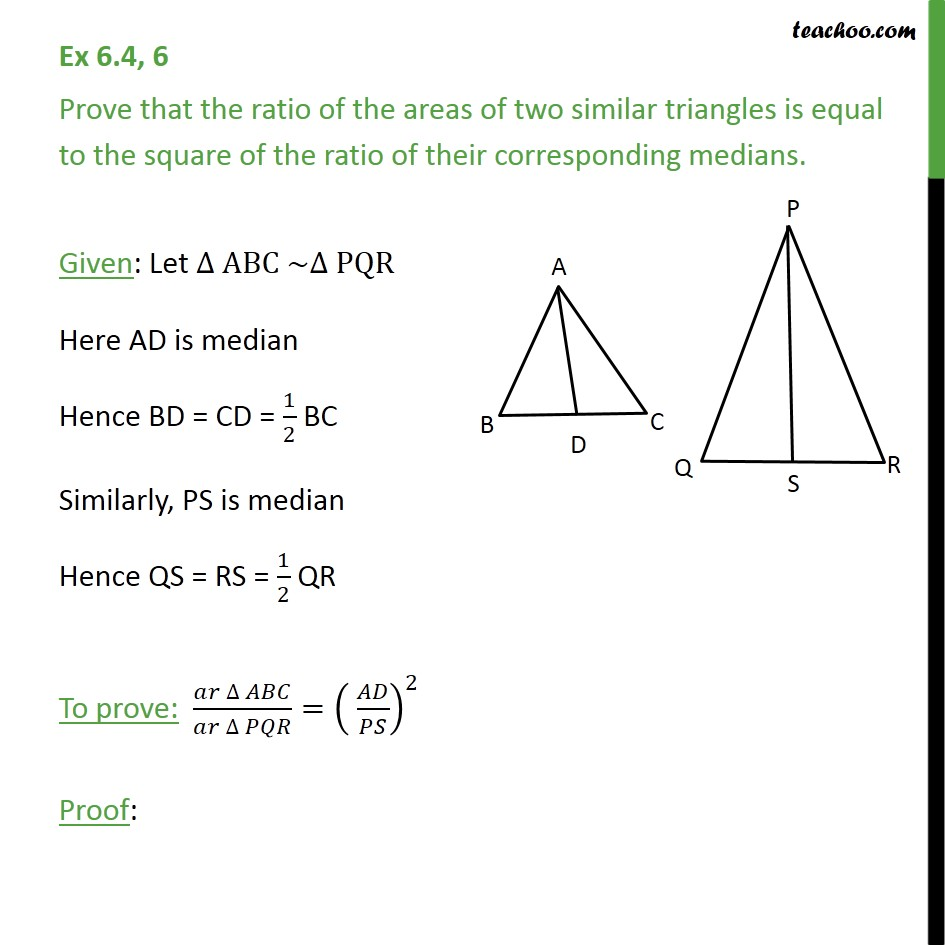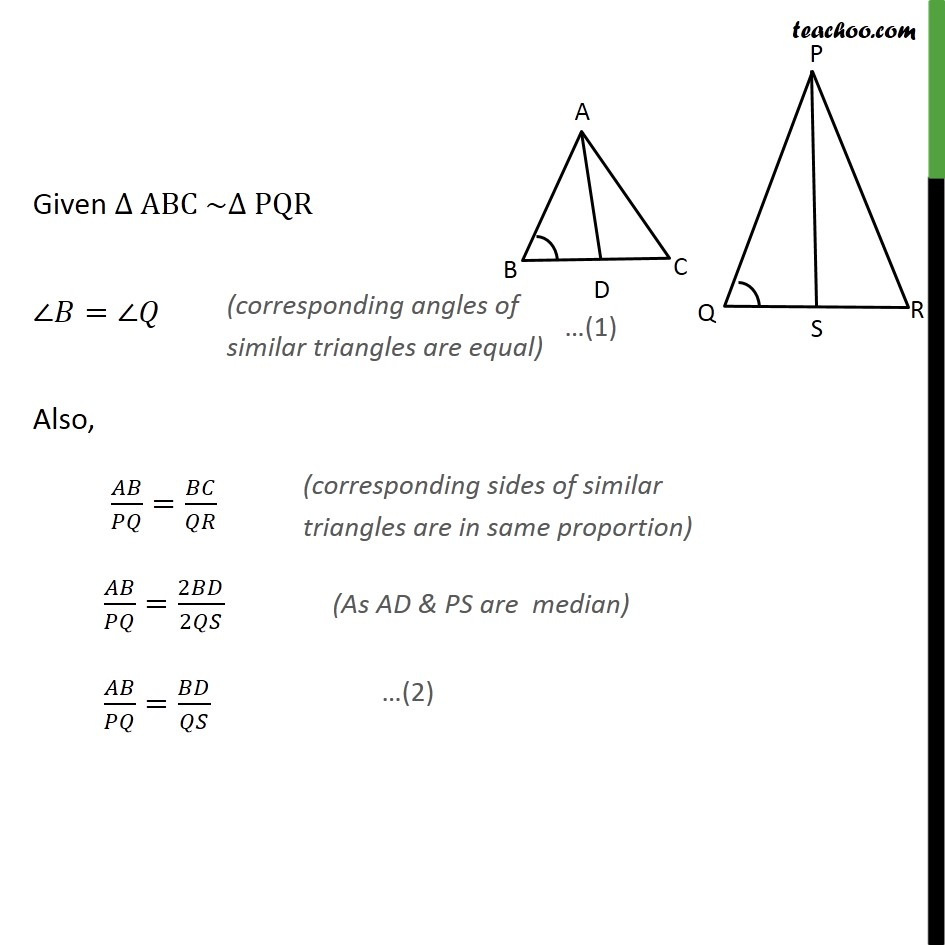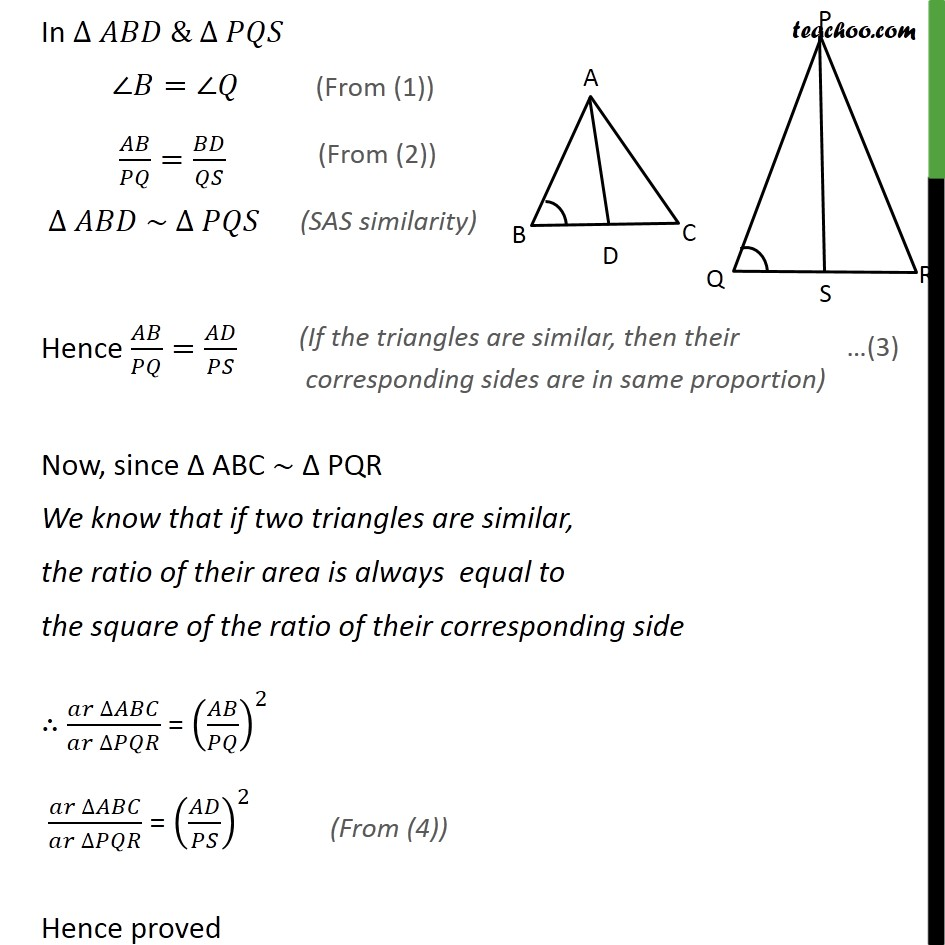Ratio of Area of Similar Triangles

Chapter 6 Class 10 Triangles
Serial order wiseLearn in your speed, with individual attention - Teachoo Maths 1-on-1 Class

### Transcript

Question 6 Exercise 6.4 Chapter 6 Class 10 CBSE NCERT Maths Prove that the ratio of the areas of two similar triangles is equal to the square of the ratio of their corresponding medians. Given: Let ABC ~ PQR Here AD is median Hence BD = CD = 1/2 BC Similarly, PS is median Hence QS = RS = 1/2 QR To prove: ( )/( )=( / )^2 Proof: Given ABC ~ PQR = Also, / = / / =2 /2 / = / In & = / = / ~ Hence / = / Now, since ABC PQR We know that if two triangles are similar, the ratio of their area is always equal to the square of the ratio of their corresponding side ( )/( ) = ( / )^2 ( )/( ) = ( / )^2 Hence proved